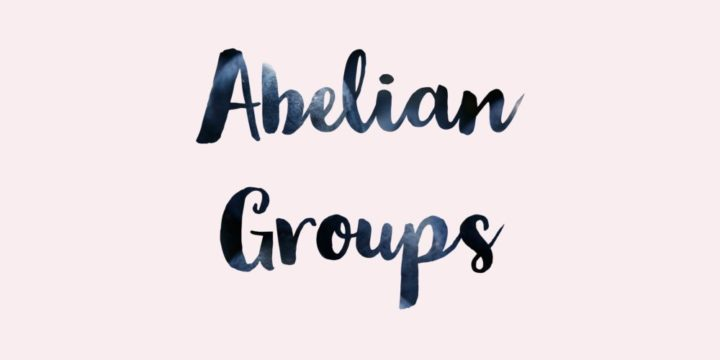# The Additive Group $\R$ is Isomorphic to the Multiplicative Group $\R^{+}$ by Exponent Function## Problem 322

Let $\R=(\R, +)$ be the additive group of real numbers and let $\R^{\times}=(\R\setminus\{0\}, \cdot)$ be the multiplicative group of real numbers.

(a) Prove that the map $\exp:\R \to \R^{\times}$ defined by
$\exp(x)=e^x$ is an injective group homomorphism.

(b) Prove that the additive group $\R$ is isomorphic to the multiplicative group
$\R^{+}=\{x \in \R \mid x > 0\}.$Add to solve later

## Proof.

### (a) Prove $\exp:\R \to \R^{\times}$ is an injective group homomorphism.

We first prove that $\exp$ is a group homomorphism.
Let $x, y \in \R$. Then we have
\begin{align*}
\exp(x+y)&=e^{x+y}\\
&=e^x e^y\\
&=\exp(x)\exp(y).
\end{align*}
Thus, the map $\exp$ is a group homomorphism.

To show that $\exp$ is injective, suppose $\exp(x)=\exp(y)$ for $x, y\in \R$.
This implies that we have
$e^{x}=e^{y},$ and thus $x=y$ by taking $\log$ of both sides.
Hence $\exp$ is an injective group homomorphism.

### (b) Prove that the additive group $\R$ is isomorphic to the multiplicative group $\R^{+}$.

Since the image of $\exp:\R \to \R^{\times}$ consists of positive numbers, we can restrict the codomain of $\exp$ to $\R^{+}$, and we have the injective homomorphism
$\exp: \R \to \R^{+}.$

It suffices to show that this homomorphism is surjective.
For any $y\in \R^{+}$, we have $\log(y)\in \R$ and
$\exp(\log(y))=e^{\log(y)}=y.$

Thus, $\exp: \R \to \R^{+}$ is a bijective homomorphism, hence isomorphism of groups.
This proves that the additive group $\R$ is isomorphic to the multiplicative group $\R^{+}$.

Note that the inverse homomorphism is given by
$\log: \R^{+} \to \R$ sending $x\in \R^{+}$ to $\log(x)$.

This is a group homomorphism since we have for $x, y \in \R^{+}$,
\begin{align*}
\log(xy)=\log(x)+\log(y)
\end{align*}
by the property of the log function.Add to solve later

### More from my site

#### You may also like...

This site uses Akismet to reduce spam. Learn how your comment data is processed.

###### More in Group Theory##### Torsion Subgroup of an Abelian Group, Quotient is a Torsion-Free Abelian Group

Let $A$ be an abelian group and let $T(A)$ denote the set of elements of $A$ that have finite order....

Close